# RD Sharma Solutions for Class 10 Maths Chapter 8 Quadratic Equations Exercise 8.11

Mensuration is also a wide scope in mathematics. In this exercise, the applications of quadratic equations for solving problems on mensuration is prominently discussed. Students facing any obstacles in solving problems can make use of the RD Sharma Solutions Class 10. The RD Sharma Solutions for Class 10 Maths Chapter 8 Quadratic Equations Exercise 8.11 PDF given below can also be used for clearing conceptual doubts related to this exercise.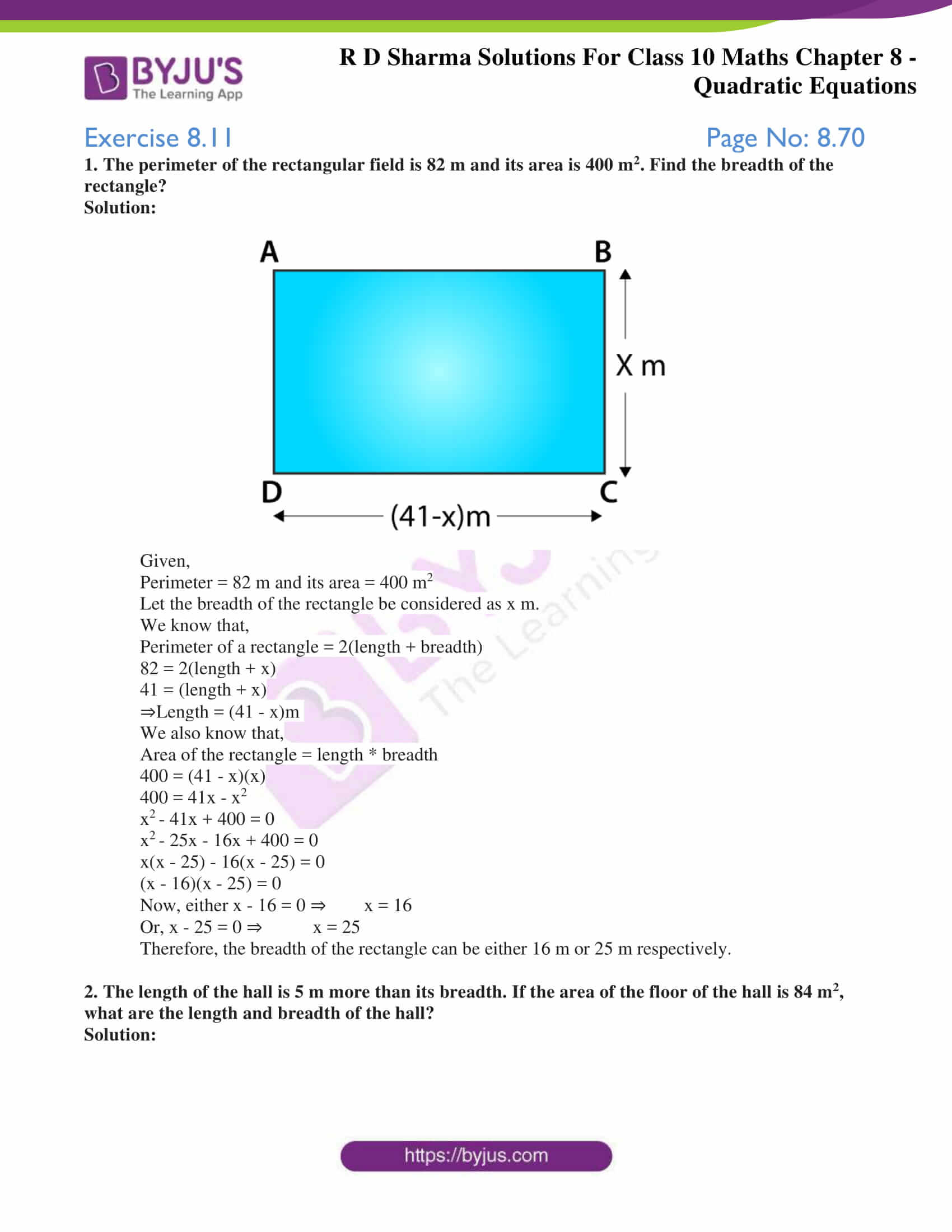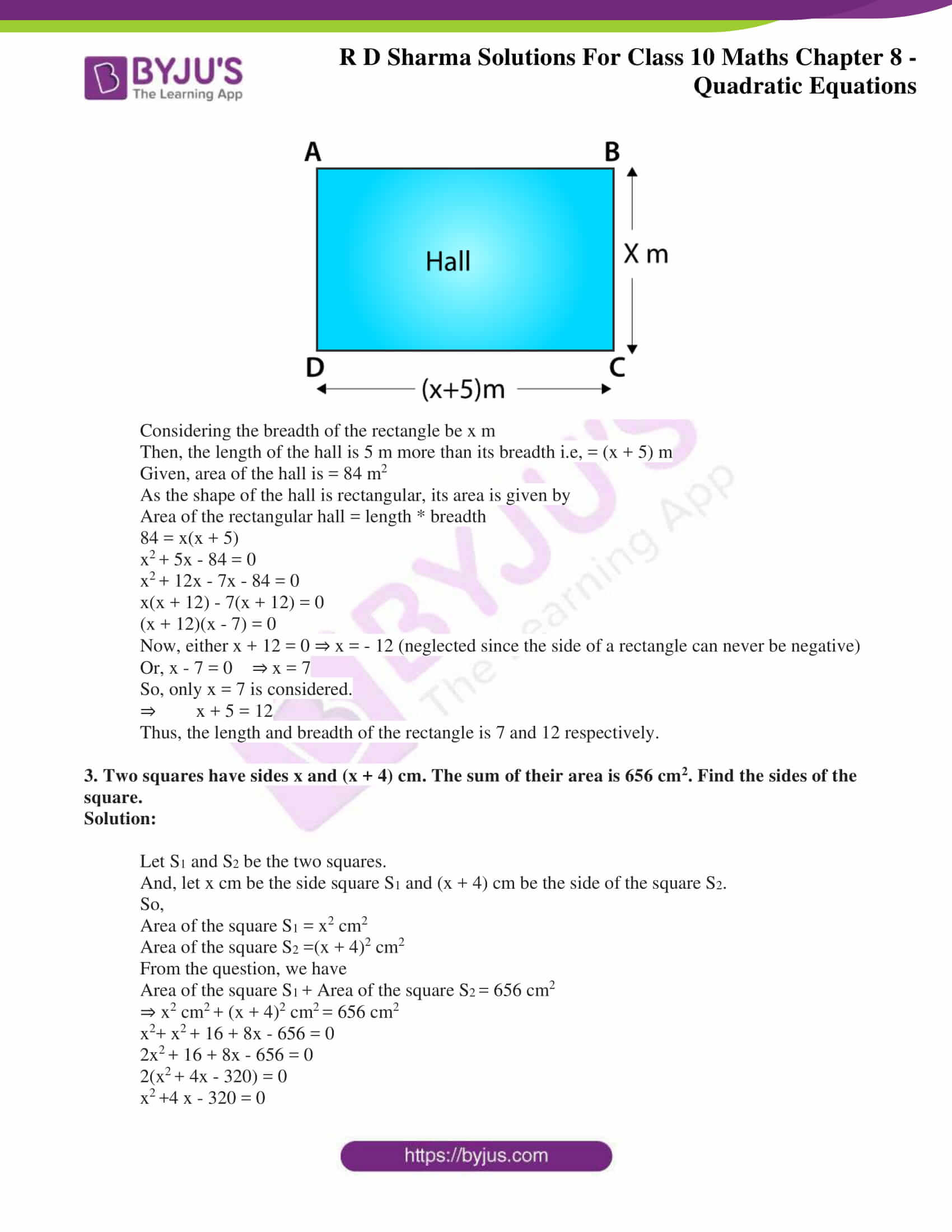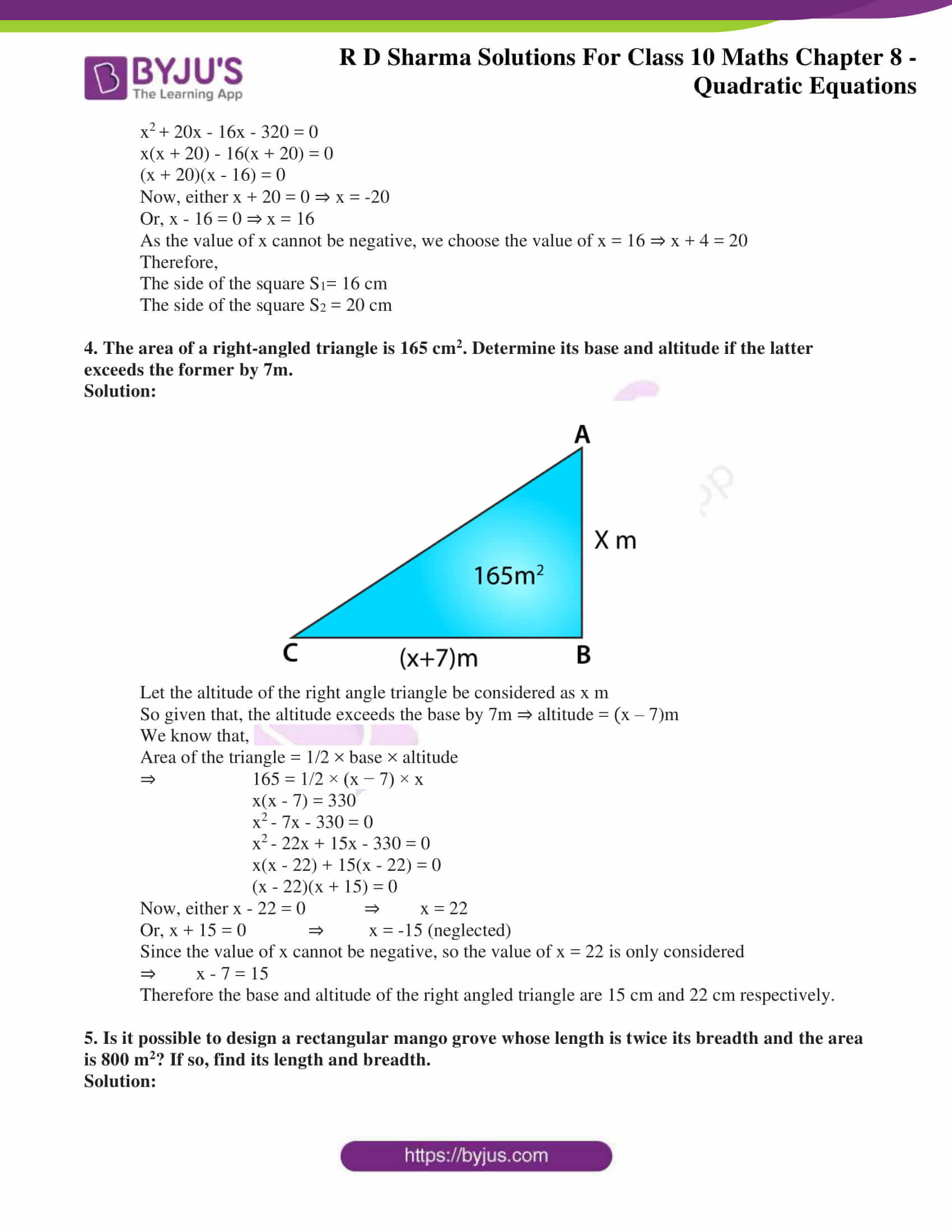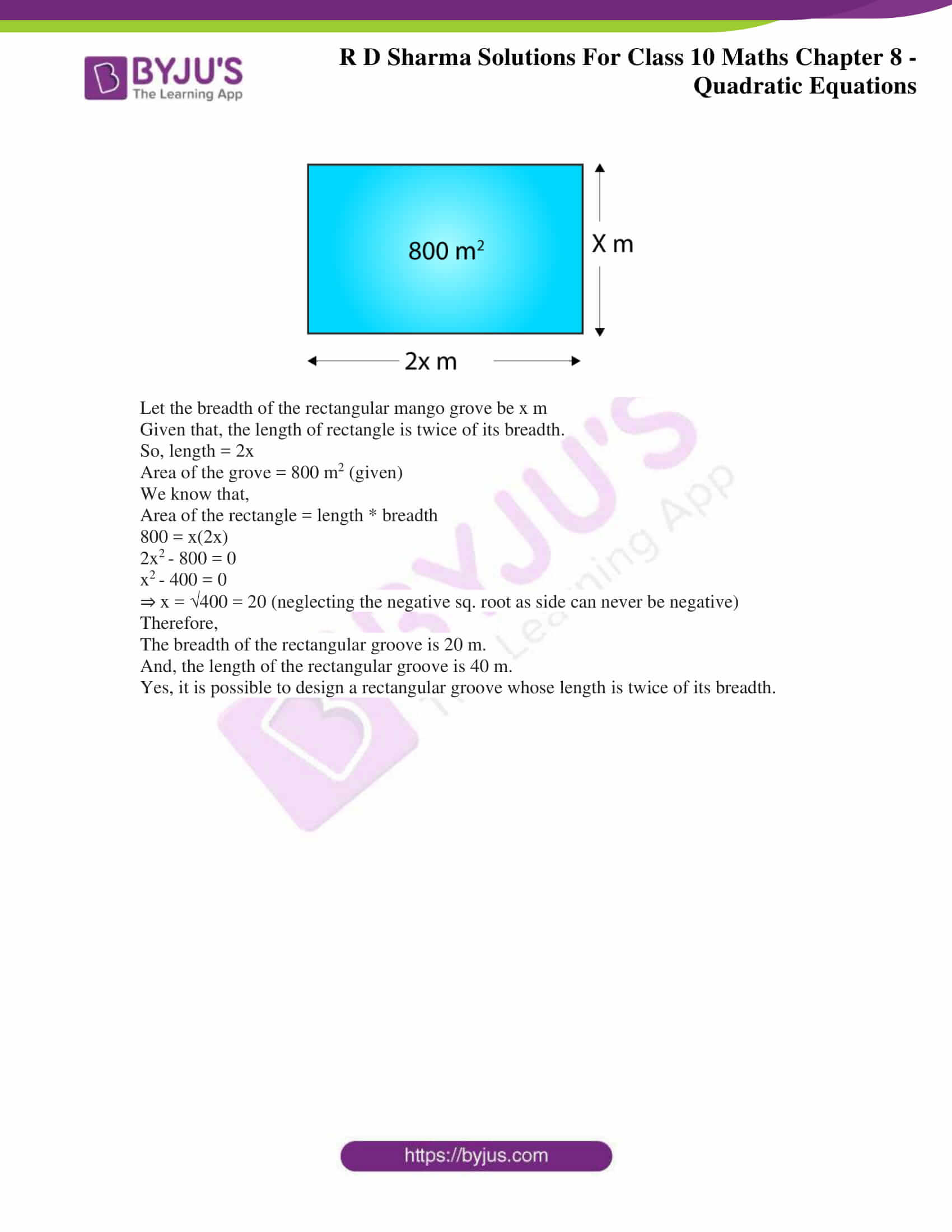### Access RD Sharma Solutions for Class 10 Maths Chapter 8 Quadratic Equations Exercise 8.11

1. The perimeter of the rectangular field is 82 m and its area is 400 m2. Find the breadth of the rectangle?

Solution: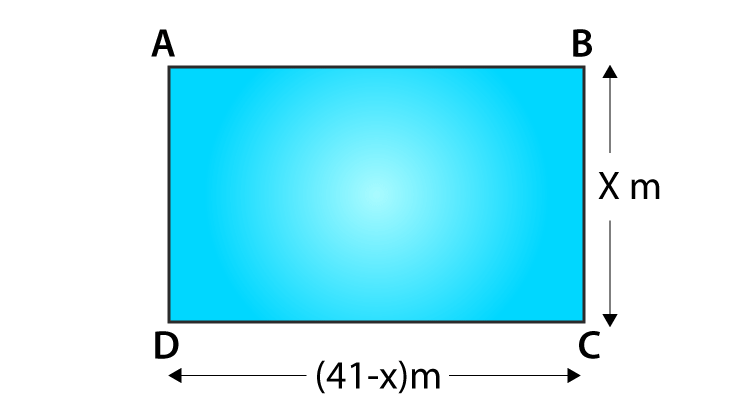Given,

Perimeter = 82 m and its area = 400 m2

Let the breadth of the rectangle be considered as x m.

We know that,

Perimeter of a rectangle = 2(length + breadth)

82 = 2(length + x)

41 = (length + x)

⇒Length = (41 – x)m

We also know that,

Area of the rectangle = length * breadth

400 = (41 – x)(x)

400 = 41x – x2

x– 41x + 400 = 0

x– 25x – 16x + 400 = 0

x(x – 25) – 16(x – 25) = 0

(x – 16)(x – 25) = 0

Now, either x – 16 = 0 ⇒ x = 16

Or, x – 25 = 0 ⇒ x = 25

Therefore, the breadth of the rectangle can be either 16 m or 25 m respectively.

2. The length of the hall is 5 m more than its breadth. If the area of the floor of the hall is 84 m2, what are the length and breadth of the hall?

Solution: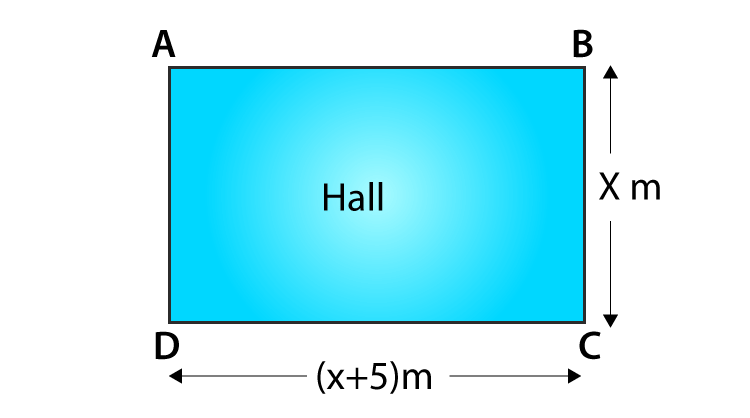Considering the breadth of the rectangle be x m

Then, the length of the hall is 5 m more than its breadth i.e, = (x + 5) m

Given, area of the hall is = 84 m2

As the shape of the hall is rectangular, its area is given by

Area of the rectangular hall = length * breadth

84 = x(x + 5)

x+ 5x – 84 = 0

x+ 12x – 7x – 84 = 0

x(x + 12) – 7(x + 12) = 0

(x + 12)(x – 7) = 0

Now, either x + 12 = 0 ⇒ x = – 12 (neglected since the side of a rectangle can never be negative)

Or, x – 7 = 0 ⇒ x = 7

So, only x = 7 is considered.

⇒ x + 5 = 12

Thus, the length and breadth of the rectangle is 7 and 12 respectively.

3. Two squares have sides x and (x + 4) cm. The sum of their area is 656 cm2. Find the sides of the square.

Solution:

Let S1 and S2 be the two squares.

And, let x cm be the side square S1 and (x + 4) cm be the side of the square S2.

So,

Area of the square S1 = x2 cm2

Area of the square S2 =(x + 4)2 cm2

From the question, we have

Area of the square S+ Area of the square S= 656 cm2

⇒ x2 cm+ (x + 4)2 cm= 656 cm2

x2+ x+ 16 + 8x – 656 = 0

2x+ 16 + 8x – 656 = 0

2(x+ 4x – 320) = 0

x+4 x – 320 = 0

x+ 20x – 16x – 320 = 0

x(x + 20) – 16(x + 20) = 0

(x + 20)(x – 16) = 0

Now, either x + 20 = 0 ⇒ x = -20

Or, x – 16 = 0 ⇒ x = 16

As the value of x cannot be negative, we choose the value of x = 16 ⇒ x + 4 = 20

Therefore,

The side of the square S1= 16 cm

The side of the square S2 = 20 cm

4. The area of a right-angled triangle is 165 cm2. Determine its base and altitude if the latter exceeds the former by 7m.

Solution: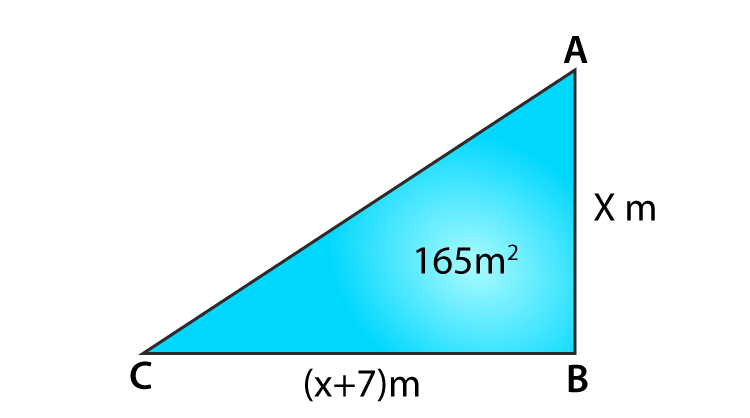Let the altitude of the right angle triangle be considered as x m

So given that, the altitude exceeds the base by 7m ⇒ altitude = (x – 7)m

We know that,

Area of the triangle = 1/2 × base × altitude

⇒ 165 = 1/2 × (x − 7) × x

x(x – 7) = 330

x– 7x – 330 = 0

x– 22x + 15x – 330 = 0

x(x – 22) + 15(x – 22) = 0

(x – 22)(x + 15) = 0

Now, either x – 22 = 0 ⇒ x = 22

Or, x + 15 = 0 ⇒ x = -15 (neglected)

Since the value of x cannot be negative, so the value of x = 22 is only considered

⇒ x – 7 = 15

Therefore the base and altitude of the right angled triangle are 15 cm and 22 cm respectively.

5. Is it possible to design a rectangular mango grove whose length is twice its breadth and the area is 800 m2? If so, find its length and breadth.

Solution: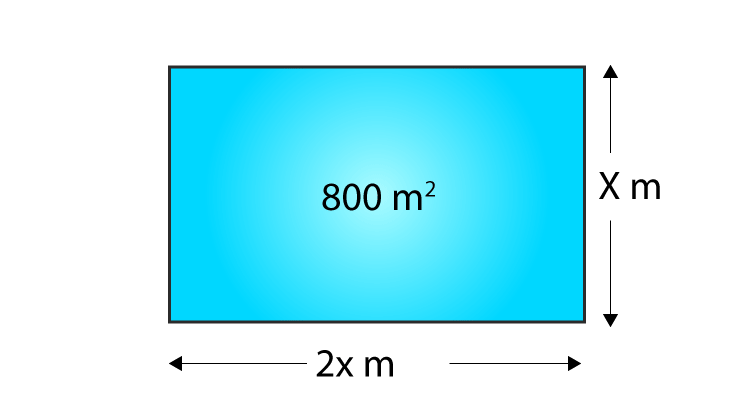Let the breadth of the rectangular mango grove be x m

Given that, the length of rectangle is twice of its breadth.

So, length = 2x

Area of the grove = 800 m2 (given)

We know that,

Area of the rectangle = length * breadth

800 = x(2x)

2x– 800 = 0

x– 400 = 0

⇒ x = √400 = 20 (neglecting the negative sq. root as side can never be negative)

Therefore,

The breadth of the rectangular groove is 20 m.

And, the length of the rectangular groove is 40 m.

Yes, it is possible to design a rectangular groove whose length is twice of its breadth.